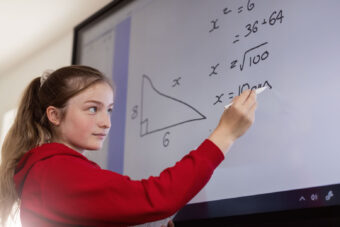SQA qualifications in Mathematics are required or desirable for courses such as Mathematics, Sciences, Accountancy, Law, Finance, Computing & IT, Architecture, Engineering Sciences and Teaching (Mathematic). Careers in banking and the actuarial profession require mathematics as well as those engaged in statistical or operational research.S1 and S2

The Curriculum for Excellence 3rd and 4th Level course aims to further develop pupil skills in Number, Money, Measure; Shape, Position, Movement and Information Handling. Throughout the course, various topics are covered and pupils take part in individual, group and paired tasks. An investigational approach is given to some of these topics.

Throughout the course, pupils can expect to work on Integers, Angles, Types of Number, Scientific Notation, Algebra, Decimals, Statistics, Percentages, Areas, Circle, Fractions, Scales & Drawing, Ratio/Proportion, Equations/Inequations, Time, Linear Patterns, Money and Pythagoras’ Theorem.

Numeracy and Problem solving are embedded into the course along with appropriate assessment. Meanwhile, pupil work is monitored continuously.

National 4 and National 5

National 4
As part of the course, pupils develop their ability to understand and apply straightforward mathematical skills in algebra, geometry, trigonometry, and statistics. Pupils use mathematical techniques and reasoning skills to solve mathematical problems, which are based on real-life situations. The course helps pupils develop skills in how to use mathematical language and explore mathematical ideas which helps them gain confidence in problem-solving, analytical skills, accuracy and interpreting, communicating and managing information in mathematical form.

National 5
The National 5 course develops pupils’ ability to understand and apply mathematical skills in algebra, geometry, trigonometry, and statistics. They are challenged to simplify and solve problems; select and apply mathematical techniques to real-life contexts and make connections and informed predictions. Pupils will use mathematical language and explore mathematical ideas. They will develop resilience and confidence in problem-solving; analytical and evaluative skills; interpreting, communicating and managing information in mathematical form.

Higher

Mathematical rigour and the ability to use precise and concise mathematical language assumes a particular importance at this stage. Candidates who complete the Higher Mathematics course successfully are expected to have a competence and a confidence in applying mathematical techniques, manipulating symbolic expressions and communicating with mathematical correctness in the solution of problems.

The course has obvious relevance for candidates with interests in fields such as commerce, engineering and science where the mathematics learned will be put to direct use.